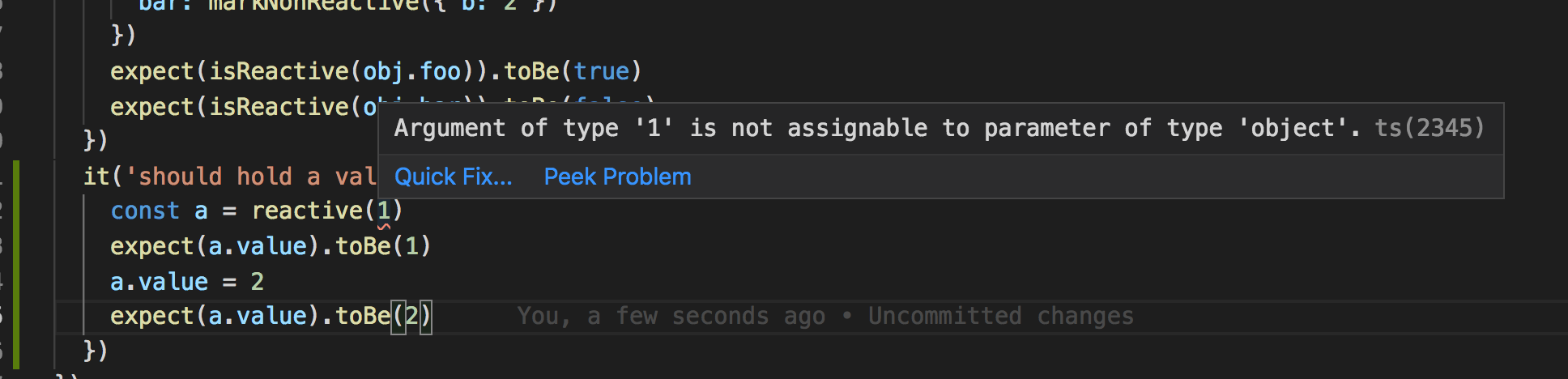# 你为什么看不懂源码之Vue 3.0 面面俱到【2】

2,450

## 是骡子是马，牵出来溜溜

`jest packages/reactivity/__tests__/reactive.spec.ts`

`````` PASS  packages/reactivity/__tests__/reactive.spec.ts
reactivity/reactive
✓ Object (6ms)
✓ Array (1ms)
✓ cloned reactive Array should point to observed values (1ms)
✓ nested reactives (5ms)
✓ observed value should proxy mutations to original (Object) (1ms)
✓ observed value should proxy mutations to original (Array) (1ms)
✓ setting a property with an unobserved value should wrap with reactive
✓ observing already observed value should return same Proxy (1ms)
✓ observing the same value multiple times should return same Proxy
✓ should not pollute original object with Proxies
✓ unwrap (1ms)
✓ non-observable values (1ms)
✓ markNonReactive (1ms)
``````

## 包罗万象

``````import { reactive, isReactive
, toRaw, markNonReactive
} from '../src/reactive'
import { mockWarn } from '@vue/runtime-test'
test('Object', () => {
const original = { foo: 1 }
// 用 reactive 包装 original，original变成了响应式数据
const observed = reactive(original)
// 这句很明显了吧，observed 不等于 original
expect(observed).not.toBe(original)
// observed 是响应式数据
expect(isReactive(observed)).toBe(true)
// original 不是响应式数据
expect(isReactive(original)).toBe(false)
// 通过响应数据 observed 拿到的值与原数据相等
expect(observed.foo).toBe(1)
// foo 这个key 值，存在于 observed 中
expect('foo' in observed).toBe(true)
// observed 的健集合与原数据相等，toEqual 是深度比较，它会比较值，而非地址
expect(Object.keys(observed)).toEqual(['foo'])
})
``````

• Object
• Array
• cloned reactive Array should point to observed values
• nested reactives
• observed value should proxy mutations to original (Object)
• observed value should proxy mutations to original (Array)
• setting a property with an unobserved value should wrap with reactive
• observing already observed value should return same Proxy
• observing the same value multiple times should return same Proxy
• should not pollute original object with Proxies
• unwrap
• non-observable values
• markNonReactive

## 优化！优化！还是TMD优化！

`在 reactive.ts` 中的 `createReactiveObject` 方法里，为什么要 `set` 两次 `toProxy.set(target, observed) toRaw.set(observed, target)`

``````// target already has corresponding Proxy
let observed = toProxy.get(target)
if (observed !== void 0) {
return observed
}
// target is already a Proxy
if (toRaw.has(target)) {
return target
}
``````

``````test('observing already observed value should return same Proxy', () => {
const original = { foo: 1 }
const observed = reactive(original)
const observed2 = reactive(observed)
expect(observed2).toBe(observed)
})
``````

## 令人困扰的 Ref

``````it('should hold a value', () => {
const a = ref(1)
expect(a.value).toBe(1)
a.value = 2
expect(a.value).toBe(2)
})
``````Typescript 的优势体现出来了，入参只支持对象，不支持基本类型！ `reactive.ts` 核心api 是 Proxy，Proxy 的传参只能是对象。如果传基本类型的话，会console

`Cannot create proxy with a non-object as target or handler at proxyMethod`

``````const convert = (val: any): any => (isObject(val) ? reactive(val) : val)

export function ref<T>(raw: T): Ref<T> {
// 如果是对象，则用 reactive 方法 包装 raw
raw = convert(raw)
// 返回一个 v 对象，在 取value 值时，调用 track 方法，在存 value 值时，调用 trigger方法
const v = {
[refSymbol]: true,
get value() {
track(v, OperationTypes.GET, '')
return raw
},
set value(newVal) {
raw = convert(newVal)
trigger(v, OperationTypes.SET, '')
}
}
return v as Ref<T>
}
``````

``````test('toRefs', () => {
const a = reactive({
x: 1,
y: 2
})

const { x, y } = toRefs(a)

expect(isRef(x)).toBe(true)
expect(isRef(y)).toBe(true)
expect(x.value).toBe(1)
expect(y.value).toBe(2)

// source -> proxy
a.x = 2
a.y = 3
expect(x.value).toBe(2)
expect(y.value).toBe(3)

// proxy -> source
x.value = 3
y.value = 4
expect(a.x).toBe(3)
expect(a.y).toBe(4)
}
``````

``````  const { x, y } = reactive({
x: 1,
y: 2
})
``````

``````
export function toRefs<T extends object>(
object: T
): { [K in keyof T]: Ref<T[K]> } {
const ret: any = {}
for (const key in object) {
ret[key] = toProxyRef(object, key)
}
return ret
}

function toProxyRef<T extends object, K extends keyof T>(
object: T,
key: K
): Ref<T[K]> {
return {
[refSymbol]: true,
get value(): any {
return object[key]
},
set value(newVal) {
object[key] = newVal
}
}
}
``````

## 未完待续

vue-next 的源码正在不断更新中，小伙伴们在看源码的过程中，要时不时pull一下，防止源码版本滞后呦......